Continuous practice using S Chand Class 10 Maths Solutions ICSE Chapter 12 Similar Triangles Ex 12(b) can lead to a stronger grasp of mathematical concepts.

## S Chand Class 10 ICSE Maths Solutions Chapter 12 Similar Triangles Ex 12(b)

Question 1.
In each question, of the three triangles, name the one that is different, i.e., not similar to the other two.Solution:
In ∆ (a), third angle = 180° – (75° + 55°) = 180° – 130° = 50°
∴ Three angles are 75°, 55°, 50°
In ∆ (b), third angle is = 180° – (55° + 80°) = 180° – 135° = 45°
Three angles are 55°, 80°, 45°
In ∆ (c), third angle is = 180° – (45° + 80°) = 180°- 125° = 55°
Three angles are 45°, 80°, 55°
We see that triangle (a) is different

(ii) In ∆ (a), third angle is = 180° – (100°+ 45°) = 180° – 145° = 35°
∴ Three angles will be 100°, 45°, 35°
In ∆ (b), third angle is = 180° – (100° + 35°) = 180° + 35° = 45°
∴ Three angles will be = 100°, 35°, 45°
In ∆ (c), third angle is = 180° – (25° + 45°) = 180° – 70° = 110°
∴ Three angles will be 110°, 25°, 45°
We see that triangle (c) is different

(iii) In ∆ (a), two sides are equal
In ∆ (b), no two sides are equal
In ∆ (c), two sides are equal
∴ ∆ (b) is different

Question 2.
Write down the ratio of the corresponding sides for each pair of triangles and check that it is the same.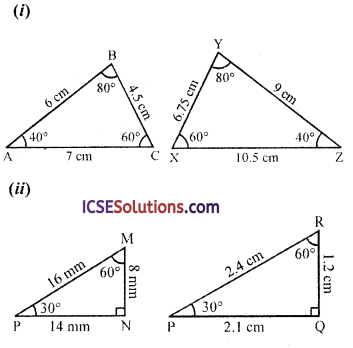Solution:
(i) In the first pair of triangle
The ratio in corresponding sides are
$$\frac{A B}{Y Z}=\frac{B C}{X Y}=\frac{A C}{X Z}$$
⇒ $$\frac{6}{9}=\frac{4.5}{6.75}=\frac{7}{10.5}$$
⇒ $$\frac{2}{3}=\frac{450}{675}=\frac{70}{105}$$
⇒ $$\frac{2}{3}=\frac{2}{3}=\frac{2}{3}$$
Yes, it is the same ratio
$$\frac{A B}{Y Z}=\frac{B C}{X Y}=\frac{A C}{X Z}=\frac{2}{3}$$

(ii) In the second pair of triangles
The ratio in the corresponding sides are
$$\frac{\mathrm{LM}}{\mathrm{RP}}=\frac{\mathrm{MN}}{\mathrm{RQ}}=\frac{\mathrm{LN}}{\mathrm{PQ}}$$
⇒ $$\frac{16}{2.4}=\frac{8}{1.2}=\frac{14}{2.1}$$
⇒ $$\frac{16 \times 10}{24}=\frac{8 \times 10}{12}=\frac{14 \times 10}{21}$$
⇒ $$\frac{20}{3}=\frac{20}{3}=\frac{20}{3}=\frac{2}{3}$$ (Dividing by 10)
Yes, it is the same ratio
∴ $$\frac{\mathrm{LM}}{\mathrm{RP}}=\frac{\mathrm{MN}}{\mathrm{RQ}}=\frac{\mathrm{LN}}{\mathrm{PQ}}=\frac{2}{3}$$Question 3.
In ∆ABCD and E are points on the sides AB and AC respectively such that DE || BC.
(i) If $$\frac{\mathrm{AD}}{\mathrm{BD}}=\frac{4}{5}$$ and EC = 2.5 cm, find AE.
(ii) If AD = 4, AE = 8, DB = x – 4, and EC = 3x – 19, find x.
(in) If AD = x, DB = x – 2, AE = x + 2 and EC = x – 1, find the vlaue of x.
(iv) If AD = 6 cm, DB = 9 cm and AE = 8 cm, find AC.
(v) If $$\frac{\mathrm{AD}}{\mathrm{BD}}=\frac{2}{3}$$ and AC = 18 cm, find AE.
(vi) If AD = 8 cm, AB = 12 cm and AE = 12 cm, find CE.
(vii) If AD = 2 cm, AB = 6 cm and AC = 9 cm, find AE.
Solution:
In ∆ABC, D and E are points on AB and AC respectively and DE || BC
(i) $$\frac { AD }{ BD }$$ = $$\frac { 4 }{ 5 }$$, EC = 2.5 cm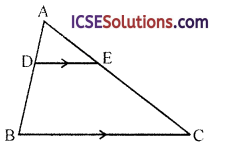In ∆ABC,
∵ DE || BC
∴ $$\frac{\mathrm{AD}}{\mathrm{BD}}=\frac{\mathrm{AE}}{\mathrm{EC}} \Rightarrow \frac{4}{5}=\frac{\mathrm{AE}}{2.5}$$
⇒ AE = $$\frac{4 \times 2.5}{5}$$ = 2 cm
∴ AE = 2 cm

(ii) In the figure, in ∆ABC, DE || BC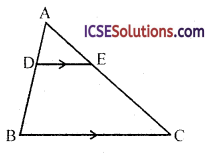∴ $$\frac{\mathrm{AD}}{\mathrm{DB}}=\frac{\mathrm{AE}}{\mathrm{EC}}$$
AD = 4, AE = 8, DB = x – 4 and EC = 3x – 19
⇒ $$\frac{4}{x-4}=\frac{8}{3 x-19}$$
⇒ 12x – 76 = 8x – 32 (By cross multiplication)
⇒ 12x – 8x = – 32 + 76
⇒ 4x = 44 ⇒ x = $$\frac { 44 }{ 4 }$$ = 11
∴ x = 11

(iii) In ∆ABC, DE || BC∴ $$\frac{\mathrm{AD}}{\mathrm{DB}}=\frac{\mathrm{AE}}{\mathrm{EC}}$$
AD = x, DB = x – 2, AE = x + 2 and EC = x – 1
∴ $$\frac{x}{x-2}=\frac{x+2}{x-1}$$
⇒ x (x – 1) = (x + 2) (x – 2) (By cross multiplication)
⇒ x² – x = x² – 4
⇒ – x = – 4
⇒ x = 4
∴ x = 4

(iv) In ∆ABC, DE || BC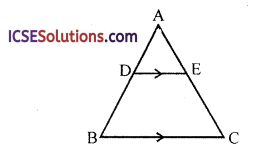∴ $$\frac{\mathrm{AD}}{\mathrm{DB}}=\frac{\mathrm{AE}}{\mathrm{EC}}$$
AD = 6 cm, DB = 9 cm, AE = 8 cm
∴ $$\frac{6}{9}=\frac{8}{\mathrm{EC}} \Rightarrow \mathrm{EC}=\frac{9 \times 8}{6}$$
⇒ EC = 12 cm
∴ AC = AE + EC = 8 + 12 = 20 cm

(v) In ∆ABC, DE || BC∴ $$\frac{\mathrm{AD}}{\mathrm{DB}}=\frac{\mathrm{AE}}{\mathrm{EC}}$$
$$\frac { AD }{ DB }$$ = $$\frac { 2 }{ 3 }$$, AC = 18 cm
Let AE = x cm
∴ EC = AC – AE = (18 -x) cm
∴ $$\frac { 2 }{ 3 }$$ = $$\frac { x }{ 18-x }$$ ⇒ 3x = 36 – 2x (By cross multiplication)
⇒ 3x + 2x = 36 ⇒ 5x = 36
⇒ x = $$\frac { 2 }{ 3 }$$ = 7.2
∴ AE = 7.2

(vi) In ∆ABC, DE || BC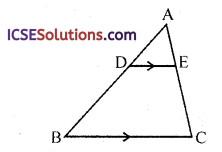∴ $$\frac { AD }{ DB }$$ = $$\frac { AE }{ EC }$$
AD = 8 cm, AB = 12 cm, AE = 12 cm DB = AB – AD = 12 – 8 = 4 cm
$$\frac { 8 }{ 4 }$$ = $$\frac { 12 }{ CE }$$ ⇒ CE = $$\frac { 4×12 }{ 8 }$$ = 6
∴ CE = 6 cm

(vii) In ∆ABC, DE || BC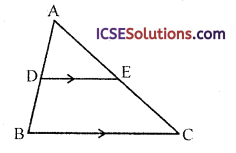∴ $$\frac{\mathrm{AD}}{\mathrm{DB}}=\frac{\mathrm{AE}}{\mathrm{EC}}$$
AD = 2 cm, AB = 6 cm, AC = 9 cm
DB = AB – AD = 6 – 2 = 4 cm
Let AE = x, then EC = AC – AE = 9 – x
∴ $$\frac{2}{4}=\frac{x}{9-x}$$ ⇒ 4x = 18 – 2x
⇒ 4x + 2x = 18
⇒ 6x = 18
⇒ x = $$\frac { 18 }{ 6 }$$ = 3
∴ AE = 3 cmQuestion 4.
In a ∆ABC, D and E are points on the sides AB and AC respectively, for each of the following cases, show that DE || BC.
(i) AD = 3, BD = 4.5, AE = 4, CE = 6.
(ii) AB = 12 cm, AD = 8 cm, AE = 12 cm and AC = 18 cm.
(iii) AB = 5.6 cm, AD = 1.4 cm, AC 7.2 cm and AE = 1.8 cm.
Solution:
(i) In ∆ABC, D and E are the points on the sides AB and AC respectively such that AD = 3, BD = 4.5, AE = 4 and CE = 6(ii) In ∆ABC, D and E are the points on the sides AB and AC respectively such that AB = 12 cm, AD = 8 cm, AE = 12 cm and AC = 18 cm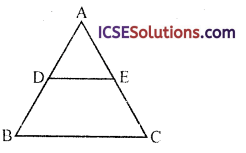∴ DB = AB – AD = 12 – 8 = 12 – 8 = 4 cm
EC = AC – AE = 18 – 12 = 6 cm
Now $$\frac{\mathrm{AD}}{\mathrm{DB}}=\frac{8}{4}=\frac{2}{1}$$
and $$\frac{\mathrm{AE}}{\mathrm{EC}}=\frac{12}{6}=\frac{2}{1}$$
∵ $$\frac{\mathrm{AD}}{\mathrm{DB}}=\frac{\mathrm{AE}}{\mathrm{EC}}$$
∴ DE || BC

(iii) In ∆ABC, D and E are the points on the sides AB and AC respectively such that AB = 5.6 cm, AD = 1.4 cm, AC = 7.2 cm and AE = 1.8 cm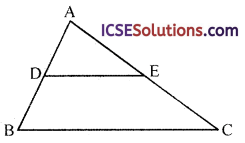∴ DB = AB – AD = 5.6 – 1.4 = 4.2 cm
EC = AC – AE = 7.2 – 1.8 = 5.4 cm
Now $$\frac{\mathrm{AD}}{\mathrm{DB}}=\frac{1.4}{4.2}=\frac{1}{3}$$
and $$\frac{\mathrm{AE}}{\mathrm{EC}}=\frac{1.8}{5.4}=\frac{1}{3}$$
∵ $$\frac{\mathrm{AD}}{\mathrm{DB}}=\frac{\mathrm{AE}}{\mathrm{EC}}$$
∴ DE || BC

Question 5.
In the figure, ABC and DEF and similar triangles, find the values of x and y, if the sides are as marked.Solution:
In the figure, ∆ABC and ∆DEF are similar
AB = x, AC = 7, BC = y
DE = 5, DF = 3 and EF = 8
∵ ∆s are similar
Corresponding sides are proportional
∴ $$\frac{\mathrm{AB}}{\mathrm{DE}}=\frac{\mathrm{AC}}{\mathrm{DF}}=\frac{\mathrm{BC}}{\mathrm{EF}}$$
$$\frac{x}{5}=\frac{7}{3}=\frac{y}{8}$$
$$\frac{x}{5}=\frac{7}{3} \Rightarrow x=\frac{5 \times 7}{3}=\frac{35}{3}=11 \frac{2}{3}$$
and $$\frac{y}{8}=\frac{7}{3} \Rightarrow y=\frac{8 \times 7}{3}=\frac{56}{3}=18 \frac{2}{3}$$

Question 6.
In the figure, ∆ABO ~ ADCO. If CD = 2 cm, AB = 3 cm, OC = 3.2 cm, OD = 2.4 cm, determine OA and OB.Solution:
∴ Their corresponding sides are proportional
∴ $$\frac{\mathrm{AB}}{\mathrm{CD}}=\frac{\mathrm{AO}}{\mathrm{OD}}=\frac{\mathrm{BO}}{\mathrm{OC}}$$
But AB = 3 cm, CD = 2 cm, OC = 3.2 cm and OD = 2.4 cm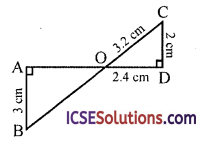Hence OA = 3.6 cm and OB = 4.8 cm

Question 7.
In the figure, ∆ABC ~ ∆ADE. If AD : DB = 2:3 and DE = 5 cm. (i) find BC (ii) if x be the length of the perpendicular from A to DE, find the length of the perpendicular from A to BC in terms of x.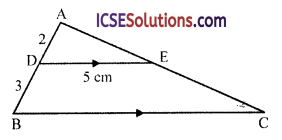Solution:
In the figure,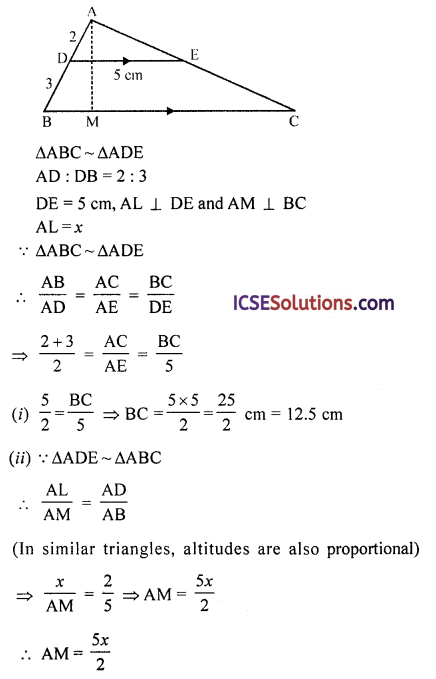Question 8.
In the figure, ABCD is a trapezium with AB parallel to DC. Given that AB = 4 cm, BC = 3 cm and CD = 6 cm.
(i) Name two triangles in the figure which are similar.
(ii) Calculate the length of EB.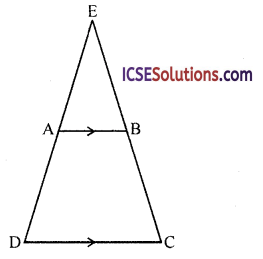Solution:
In trapezium ABCD, AB || DC
AB = 4 cm, BC = 3 cm and CD = 6 cm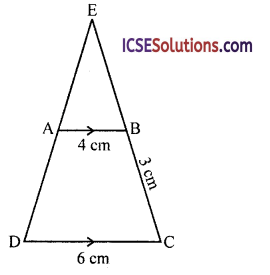(i) In the figure,
∆EDC and ∆EAB
∠E = ∠E (common)
∠EDC = ∠EAB (corresponding angles)
∠ECD = ∠EBA (corresponding angles)
∴ ∆EDC ~ ∆EAB

(ii) Let EB = x, then EC = x + 3
∵ ∆EAB ~ ∆EDC
∴ $$\frac{E B}{E C}=\frac{A B}{D C}$$
⇒ $$\frac{x}{x+3}=\frac{4}{6}$$ ⇒ 6x = 4x + 12
⇒ 6x – 4x = 12 ⇒ 2x = 12 12
⇒ x = $$\frac { 12 }{ 2 }$$ = 6
∴ EB = 6 cmQuestion 9.
A man of height 1.8 m is standing 5 m away from a lamp post and observes that the length of his shadow is 1.5 m. Find the height of the lamp post.
Solution:
∵ The mean and the lamp post are perpendicular on the ground
Height of man PQ = 1.8 m
and his shadow QN = 1.5 m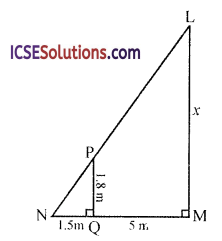Let LM be the lamp post and LM = x m
Now in ∆LMN
∵ PQ || LM
(both perpendiculars on the same line)
∴ ∆PNQ ~ ∆LNM
∵ $$\frac{\mathrm{PQ}}{\mathrm{LM}}=\frac{\mathrm{NQ}}{\mathrm{NM}}$$
⇒ $$\frac{1.8}{x}=\frac{1.5}{1.5+5} \Rightarrow \frac{1.8}{x}=\frac{1.5}{6.5}$$
⇒ x = $$\frac{1.8 \times 6.5}{1.5}$$
∴ Height of the lamp post = 7.8 m

Question 10.
In figure, ACE and BCD are two straight lines.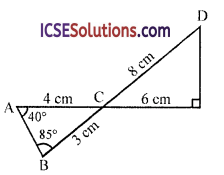∠A = 40° and ∠B = 85°. Using the measurements given in the figure, complete the following true statements
(i) Triangles ABC and CDE are similar because ……… and ………
(ii) The size of ∠D is ………
(iii) If AB = x cm, then ED = ………
Solution:
Two lines ACE and BCD intersect each other at C
AC = 4 cm, CE = 6 cm
BC = 3 cm and CD = 8 cm
(i) In ∆ABC and ∆CDE
$$\frac{\mathrm{BC}}{\mathrm{AC}}=\frac{3}{4} \text { and } \frac{\mathrm{CE}}{\mathrm{CD}}=\frac{6}{8}=\frac{3}{4}$$
∠ACB = ∠DCE (vertically opposite angles)
∴ ∆ABC ~ ACDE because $$\frac{B C}{A C}=\frac{C E}{C D}=\frac{3}{4}$$ and ∠ACB = ∠DCE

(ii) ∠D = ∠A = 40° (corresponding angles)

(iii) AB = x cm
and $$\frac{\mathrm{AC}}{\mathrm{CD}}=\frac{\mathrm{AB}}{\mathrm{DE}}=\frac{4}{8}=\frac{1}{2}$$
⇒ $$\frac{x}{\mathrm{DE}}=\frac{1}{2}$$
⇒ DE = 2x
∴ DE = 2x

Question 11.
The triangle ABC is right-angled at C. From P, a point on the hypotenuse, PQ is drawn parallel to AC cutting BC at Q. If AC 2.5 cm, BC = 6 cm and PQ = 1 cm, find
(i) BQ
(ii) BP
Solution:
In right angle ∆ABC, ∠C = 90
P is any point on ABFrom P, PQ is drawn parallel to AC which meets BC at Q
AC = 2.5 cm, BC = 6 cm, PQ = 1 cm
In ∆ABC
∵ PQ || AC

(i) ∴ ∆ABC ~ APBQ
$$\frac{\mathrm{AC}}{\mathrm{PQ}}=\frac{\mathrm{BC}}{\mathrm{BQ}} \Rightarrow \frac{2.5}{1}=\frac{6}{\mathrm{BQ}}$$
⇒ BQ = $$\frac{6 \times 1}{2.5}=\frac{6}{2.5}$$ = 2.4 cm

(ii) In right ∆PQB (∵ ∠Q = ∠C = 90°)
BP² = PQ² + BQ²
= (1)² + (2.4)²
= 1 + 5.76
= 6.75
= (2.6)²
BP = 2.6 cm

Question 12.
State whether the following statement is true or false, briefly giving the reasons. If two angles of one triangle are 72° and 80° respectively and that of another triangle are 28° and 72° respectively, then the triangles are similar.
Solution:
Let in one triangle ABC,
∠A = 72°, ∠B = 80°
Then ∠C =180°- (72° + 80°) = 180° – 152° = 28°
and in the other triangle PQB
∠P = 28°, ∠Q = 72°
∴ ZR = 180° – (28° + 72°) = 180° – 100° = 80°
∵ ∠A = ∠Q, ∠B = ZR and ∠C = ∠P
∴ ∆ABC ~ APQR (AAA axiom)Question 13.
In figure, ∠ADE = ∠B, show that ∆ABC ~ ∆ADE. If AD = 2.7 cm, AE = 2.5 cm, BE = 1.1 cm and BC = 5.2 cm, find DE.Solution:
In the figure, AD = 2.7 cm, AE = 2.5 cm, BE = 1.1 cm and BC = 5.2 cm
∠A = ∠A (common)
∴ ∆ABC ~ ∆ADE (AA axiom)

(ii) ∴ $$\frac{B C}{D E}=\frac{A B}{A D}=\frac{A C}{A E}$$
(corresponding sides are proportional)
⇒ $$\frac{5.2}{\mathrm{DE}}=\frac{(1.1+2.5)}{2.7}$$
⇒ $$\frac{5.2}{\mathrm{DE}}=\frac{3.6}{2.7} \Rightarrow \mathrm{DE}=\frac{5.2 \times 2.7}{3.6}$$ = 3.9
Hence DE = 3.9 cm

Question 14.
In figure, AB, EF and CD are parallel lines. Given that AB = 15 cm, EG = 5 cm, GC = 10 cm and DC = 18 cm. Calculate EF and AC.Solution:
In the figure, ABC is a triangle AB, EF and CD are parallel line
AB = 15 cm, EG = 5 cm, BC = 10 cm and DC =18 cm
We have to find EF and AC
(i) In ∆EFG and ∆CGD
∠EGF = ∠CGD
(vertically opposite angles)
∠EFG = ∠GDC (alternate angles)
∴ ∆EFG ~ ∆CGD (∆A axiom)
∴ $$\frac{E G}{C G}=\frac{E F}{C D}$$
(corresponding sides are proportional)
⇒ $$\frac{5}{10}=\frac{\mathrm{EF}}{18} \Rightarrow \mathrm{EF}=\frac{5 \times 18}{10}$$ = 9
∴ EF = 9 cm

(ii) Similarly in ∆ABC and AECF
∠C = ∠C (common)
∠CAB = ∠CEF (corresponding angles)
∆ABC ~ AECF (AA axiom)
∴ $$\frac{A C}{E C}=\frac{A B}{E F} \Rightarrow \frac{A C}{10+5}=\frac{15}{9} \Rightarrow \frac{A C}{15}=\frac{15}{9}$$
⇒ AC = $$\frac{15 \times 15}{9}=\frac{225}{9}$$ = 25
∴ AC = 25 cm

Question 15.
Calculate the other sides of a triangle whose shortest side is 6 cm and which is similar to a triangle whose sides are 4, 7 and 8 cm.
Solution:
Let ∆ABC ~ ∆PQR and let
in ∆PQR, PQ = 4 cm, QR = 7 cm and RP = 8 cm
and let in ∆ABC, AB = 6 cm, BC = x and CA = y
Then $$\frac{P Q}{A B}=\frac{Q R}{B C}=\frac{R P}{C A}$$ (∵ As are similar)
⇒ $$\frac{4}{6}=\frac{7}{x}=\frac{8}{y}$$
Now $$\frac{7}{x}=\frac{4}{6}$$ ⇒ x = $$\frac{7 \times 6}{4}=\frac{21}{2}$$ = 10.5
and $$\frac{8}{y}=\frac{4}{6} \Rightarrow y=\frac{6 \times 8}{4}$$ = 12
∴ Other two corresponding sides are 10.5 cm and 12 cm

Question 16.
In ∆ABC, DE || BC
(a) If AD = 3 cm, DB = 4 cm, EC = 12 cm, findAE.
(b) If AE = 2.7 cm, EC = 4.5 cm, AD = 2.4 cm, find BD.
(c) If AD = 2.6 cm, DB = 6.5 cm and AE = 3 cm, find EC.
(d) If AB = 6 cm, AD = 2 cm and AC = 9 cm, calculate the length of CE.Solution:
(a) In ∆ABC, DE || CB
Now AD = 3 cm, DB = 4 cm, EC = 12 cm,
Let AE = x cm
∵ In ∆ABC, DE || BC
$$\frac{\mathrm{AD}}{\mathrm{DB}}=\frac{\mathrm{AE}}{\mathrm{EC}} \Rightarrow \frac{3}{4}=\frac{\mathrm{AE}}{12}$$
⇒ $$\frac{3}{4}=\frac{x}{12} \Rightarrow x=\frac{3 \times 12}{4}$$ = 9
∴ AE = 9 cm

(b) In ∆ABC, DE || BC
∴ $$\frac{A D}{D B}=\frac{A E}{E C}$$
⇒ $$\frac{2.4}{\mathrm{BD}}=\frac{2.7}{4.5}$$
⇒ BD = $$\frac{2.4 \times 4.5}{2.7}$$
∴ BD = 4 cm

(c) In ∆ABC, DE || BC
∴ $$\frac{A D}{D B}=\frac{A E}{E C}$$
⇒ $$\frac{2.6}{6.5}=\frac{3}{\mathrm{EC}} \Rightarrow \mathrm{EC}=\frac{6.5 \times 3}{2.6}$$
⇒ EC = $$\frac { 15 }{ 2 }$$ = 7.5
∴ EC = 7.5 cm

(d) In ∆ABC, DE || BC
∴ $$\frac{A D}{D B}=\frac{A E}{A C}$$
⇒ $$\frac{2}{6}=\frac{A E}{9}$$
⇒ AE = $$\frac { 2×9 }{ 6 }$$ = 3